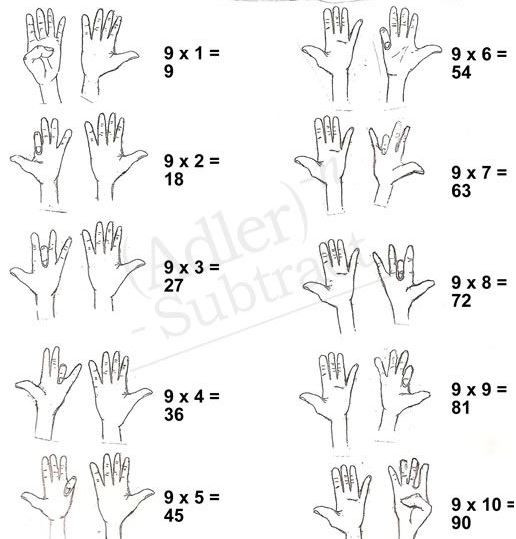Search

# Helping Your Student Memorize the Multiplication Chart

Hope you are persuaded by our earlier blog that memorizing the multiplication chart is an important building block for a solid math education. Here are some simple tricks and fun facts for helping your student memorize the multiplication chart.

When asking your student to memorize the multiplication chart, we should give her something that looks easy rather than daunting. Why do you need a crowded square chart when you know a x b = b x a ? The square chart contains too many duplicates. Something simpler such as the triangle multiplication chart below contains as much information as a square one, but looks a lot less messy and intimidating.

You can help the student memorize by asking her to do one column a day. Or one column every two days, or one column a week. Whatever it takes. She will be able to do it before the end of her third grade.

You can also point out to him the fun facts that may be obvious to an adult but may not to a young student, such as:

• All results in the “2x” column are even numbers. From one row to the next, you add the result of the first row by 2 and get the result of the next row. For example, if you already know 2x5=10, then you get 2x6 by adding 2 to 10, and you will get 12. Another easy way to arrive at any number times 2 is to add the number to itself, as is by definition.

• In the “3x” column, the sum of all digits of a multiple of 3 is itself a multiple of 3. For example, the number 24, if you add all digits of 24, you get 2+4=6 which is a multiple of 3. This is true for all multiples of 3. From one row to the next, you add the result of the first row by 3 and get the result of the next row.

• All results in the “4x” column are even numbers. From one row to the next, you add the result of the first row by 4. All results of this column are even numbers

• All results in the “5x” column ends with either number 5 or number 0. In other words, all multiples of 5 ends with 5 or 0. An even number multiple of 5 ends with 0. For example, 5x4, 5x6, 5x8 all end with 0 (5x4=4x5). An odd number multiple of 5 ends with 5. For example, 5x3, 5x5, 5x7The Almost “Magical” Times 9 Trick:

2. For 9 x 2, bend your 2nd finger down.

3. You have 1 finger in front of the bent finger and 8 after it. Thus 9 x 2 = 18.

4. This works for 9 times anything up to 10: 9 x 3, bend the 3rd finger, 9 x 4, bend the 4th finger, and so on.